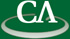### Sparse polynomial interpolation.

#### Michael Monagan, Department of Mathematics, Simon Fraser University.

Tuesday June 28th, 2011, K9509, 9:30-11:30am.

```Abstract:

Consider the problem of computing the GCD G of A and B in Zp[x0,x1,...xn].
Let d be the degree of G and t be the number of non-zero terms of G.

In the first lecture, we present Brown's modular GCD algorithm from 1971.
It interpolates the variables sequentially using dense Newton interpolation.
It needs O(d^n) univariate images to interpolate G.
For sparse polynomials like x0^d + x1^d + ... + xn^d it is inefficient.

In the second lecture, we present Zippel's sparse interpolation from 1979
which requires O(ndt) images to interpolate G and the Ben-Or/Tiwari sparse
interpolation algorithm from 1988 which requires O(T) images to interpolate G
where T is a term bound on t.

```